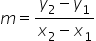Mathematics
Easy

Question

# Write the equation in point-slope form for the line that contains the points(0, -2) and (3, 4)

## y - 5 = 2(x + 3)y + 5 = 2(x - 3)y - 3 = 2(x + 5)y + 3 = 2(x - 5)Hint:

## The correct answer is: y + 5 = 2(x - 3)

### The points (0, -2) and (3, 4).Slope formulaStep 1 : Substitute points we get slope as4 - (-2) / 3 - 0= 6 / 3= 2m = 2 , is our slopeStep 2 :Point slope form y - y1 = m (x - x1)Substitute point (3, 4) and m value.y -4 = 2 (x -3). is the final equation that we get .

the equation in point slope form

y - y1 = m( x-x1)

where### Related Questions to study#### With Turito Foundation.#### Get an Expert Advice From Turito.